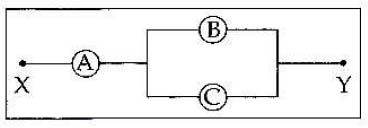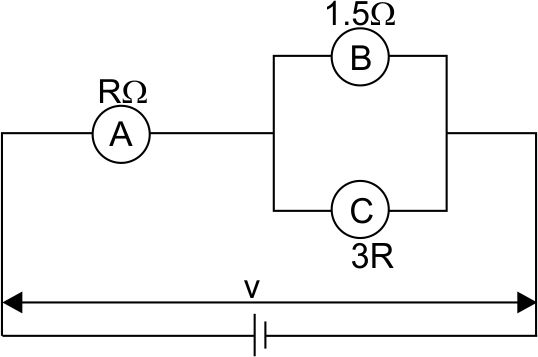#### A, B and C are voltmeters of resistance R, 1.5R and 3R respectively as shown in the figure. When some potential difference is applied between X and Y, the voltmeter readings are VA, VB and VC respectively. Then:Option 1)Option 2)Option 3)Option 4)As we learnt in

Potential difference -

The voltage accross the terminals of a cell when it is supplying current to external resistance is known as Potential Difference .

- whereinand

In series Grouping -- whereinEquivalent ResistanceOption 1)Incorrect

Option 2)Incorrect

Option 3)Correct

Option 4)Incorrect

#### Plabita